# TF-IDF简单介绍

• A+

## TF-IDF含义

TF-IDF（term frequency–inverse document frequency）是一种用于信息检索与数据挖掘的常用加权技术。

• TF意思是词频(Term Frequency)
• IDF意思是逆文本频率指数(Inverse Document Frequency)

### TF-IDF计算公式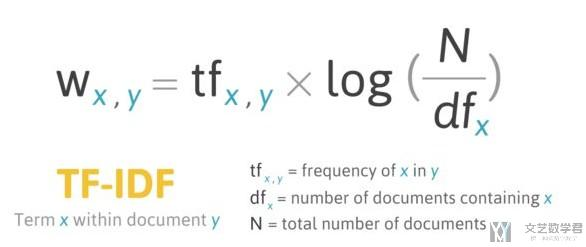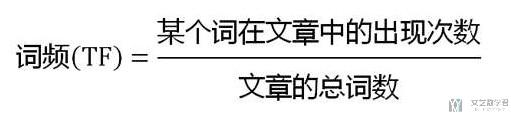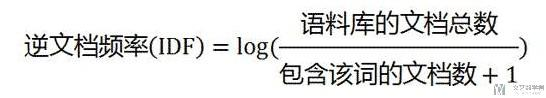TF的计算方式(详细) :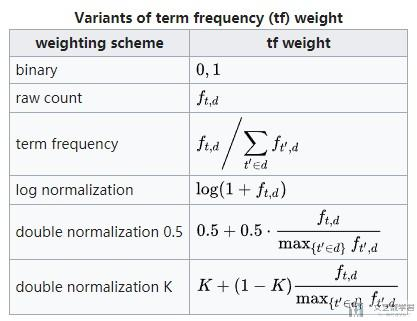IDF的计算方式(详细) :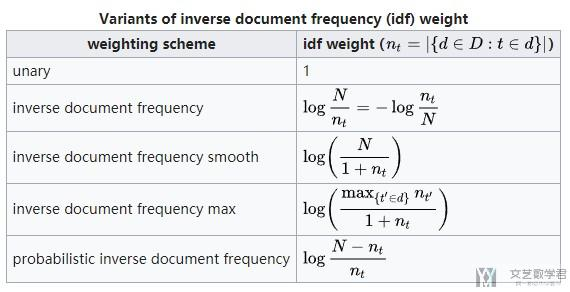## TF-IDF实际计算

### 使用sklearn计算TF-IDF

1. df = pd.DataFrame({'docId': [1,2,3], 'sent': ['我 们 好','你 们 好', '大 家 好']})
2. df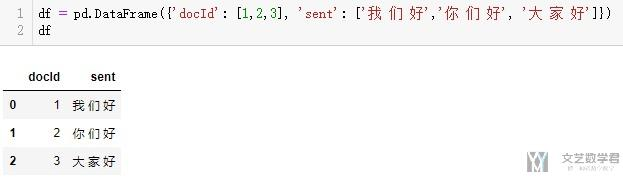• token_pattern(默认参数) : r"(?u)\b\w\w+\b"
• token_pattern(修改之后) : r"(?u)\b\w+\b"

1. v = TfidfVectorizer(token_pattern=r"(?u)\b\w+\b")
2. x = v.fit_transform(df['sent'])
3. x.toarray() # 3*7(三句话, 一共六个单词)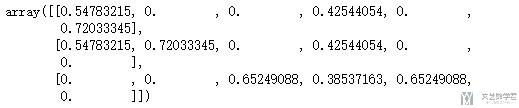1. v.get_feature_names()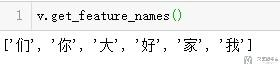• 微信公众号
• 关注微信公众号
•• QQ群
• 我们的QQ群号
•